## 2.5  Systems with Generic Operations

In the previous section, we saw how to design systems in which data objects can be represented in more than one way. The key idea is to link the code that specifies the data operations to the several representations by means of generic interface procedures. Now we will see how to use this same idea not only to define operations that are generic over different representations but also to define operations that are generic over different kinds of arguments. We have already seen several different packages of arithmetic operations: the primitive arithmetic (+, -, *, /) built into our language, the rational-number arithmetic (add-rat, sub-rat, mul-rat, div-rat) of section 2.1.1, and the complex-number arithmetic that we implemented in section 2.4.3. We will now use data-directed techniques to construct a package of arithmetic operations that incorporates all the arithmetic packages we have already constructed.

Figure 2.23 shows the structure of the system we shall build. Notice the abstraction barriers. From the perspective of someone using ``numbers,'' there is a single procedure add that operates on whatever numbers are supplied. Add is part of a generic interface that allows the separate ordinary-arithmetic, rational-arithmetic, and complex-arithmetic packages to be accessed uniformly by programs that use numbers. Any individual arithmetic package (such as the complex package) may itself be accessed through generic procedures (such as add-complex) that combine packages designed for different representations (such as rectangular and polar). Moreover, the structure of the system is additive, so that one can design the individual arithmetic packages separately and combine them to produce a generic arithmetic system.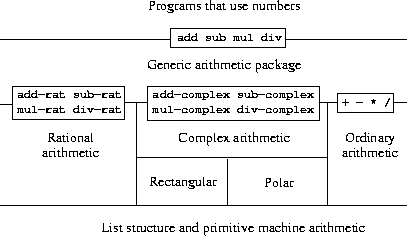### 2.5.1  Generic Arithmetic Operations

The task of designing generic arithmetic operations is analogous to that of designing the generic complex-number operations. We would like, for instance, to have a generic addition procedure add that acts like ordinary primitive addition + on ordinary numbers, like add-rat on rational numbers, and like add-complex on complex numbers. We can implement add, and the other generic arithmetic operations, by following the same strategy we used in section 2.4.3 to implement the generic selectors for complex numbers. We will attach a type tag to each kind of number and cause the generic procedure to dispatch to an appropriate package according to the data type of its arguments.

The generic arithmetic procedures are defined as follows:

(define (sub x y) (apply-generic 'sub x y))
(define (mul x y) (apply-generic 'mul x y))
(define (div x y) (apply-generic 'div x y))

We begin by installing a package for handling ordinary numbers, that is, the primitive numbers of our language. We will tag these with the symbol scheme-number. The arithmetic operations in this package are the primitive arithmetic procedures (so there is no need to define extra procedures to handle the untagged numbers). Since these operations each take two arguments, they are installed in the table keyed by the list (scheme-number scheme-number):

(define (install-scheme-number-package)
(define (tag x)
(attach-tag 'scheme-number x))
(lambda (x y) (tag (+ x y))))
(put 'sub '(scheme-number scheme-number)
(lambda (x y) (tag (- x y))))
(put 'mul '(scheme-number scheme-number)
(lambda (x y) (tag (* x y))))
(put 'div '(scheme-number scheme-number)
(lambda (x y) (tag (/ x y))))
(put 'make 'scheme-number
(lambda (x) (tag x)))
'done)

Users of the Scheme-number package will create (tagged) ordinary numbers by means of the procedure:

(define (make-scheme-number n)
((get 'make 'scheme-number) n))

Now that the framework of the generic arithmetic system is in place, we can readily include new kinds of numbers. Here is a package that performs rational arithmetic. Notice that, as a benefit of additivity, we can use without modification the rational-number code from section 2.1.1 as the internal procedures in the package:

(define (install-rational-package)
;; internal procedures
(define (numer x) (car x))
(define (denom x) (cdr x))
(define (make-rat n d)
(let ((g (gcd n d)))
(cons (/ n g) (/ d g))))
(make-rat (+ (* (numer x) (denom y))
(* (numer y) (denom x)))
(* (denom x) (denom y))))
(define (sub-rat x y)
(make-rat (- (* (numer x) (denom y))
(* (numer y) (denom x)))
(* (denom x) (denom y))))
(define (mul-rat x y)
(make-rat (* (numer x) (numer y))
(* (denom x) (denom y))))
(define (div-rat x y)
(make-rat (* (numer x) (denom y))
(* (denom x) (numer y))))
;; interface to rest of the system
(define (tag x) (attach-tag 'rational x))
(lambda (x y) (tag (add-rat x y))))
(put 'sub '(rational rational)
(lambda (x y) (tag (sub-rat x y))))
(put 'mul '(rational rational)
(lambda (x y) (tag (mul-rat x y))))
(put 'div '(rational rational)
(lambda (x y) (tag (div-rat x y))))

(put 'make 'rational
(lambda (n d) (tag (make-rat n d))))
'done)
(define (make-rational n d)
((get 'make 'rational) n d))

We can install a similar package to handle complex numbers, using the tag complex. In creating the package, we extract from the table the operations make-from-real-imag and make-from-mag-ang that were defined by the rectangular and polar packages. Additivity permits us to use, as the internal operations, the same add-complex, sub-complex, mul-complex, and div-complex procedures from section 2.4.1.

(define (install-complex-package)
;; imported procedures from rectangular and polar packages
(define (make-from-real-imag x y)
((get 'make-from-real-imag 'rectangular) x y))
(define (make-from-mag-ang r a)
((get 'make-from-mag-ang 'polar) r a))
;; internal procedures
(make-from-real-imag (+ (real-part z1) (real-part z2))
(+ (imag-part z1) (imag-part z2))))
(define (sub-complex z1 z2)
(make-from-real-imag (- (real-part z1) (real-part z2))
(- (imag-part z1) (imag-part z2))))
(define (mul-complex z1 z2)
(make-from-mag-ang (* (magnitude z1) (magnitude z2))
(+ (angle z1) (angle z2))))
(define (div-complex z1 z2)
(make-from-mag-ang (/ (magnitude z1) (magnitude z2))
(- (angle z1) (angle z2))))
;; interface to rest of the system
(define (tag z) (attach-tag 'complex z))
(lambda (z1 z2) (tag (add-complex z1 z2))))
(put 'sub '(complex complex)
(lambda (z1 z2) (tag (sub-complex z1 z2))))
(put 'mul '(complex complex)
(lambda (z1 z2) (tag (mul-complex z1 z2))))
(put 'div '(complex complex)
(lambda (z1 z2) (tag (div-complex z1 z2))))
(put 'make-from-real-imag 'complex
(lambda (x y) (tag (make-from-real-imag x y))))
(put 'make-from-mag-ang 'complex
(lambda (r a) (tag (make-from-mag-ang r a))))
'done)

Programs outside the complex-number package can construct complex numbers either from real and imaginary parts or from magnitudes and angles. Notice how the underlying procedures, originally defined in the rectangular and polar packages, are exported to the complex package, and exported from there to the outside world.

(define (make-complex-from-real-imag x y)
((get 'make-from-real-imag 'complex) x y))
(define (make-complex-from-mag-ang r a)
((get 'make-from-mag-ang 'complex) r a))

What we have here is a two-level tag system. A typical complex number, such as 3 + 4i in rectangular form, would be represented as shown in figure 2.24. The outer tag (complex) is used to direct the number to the complex package. Once within the complex package, the next tag (rectangular) is used to direct the number to the rectangular package. In a large and complicated system there might be many levels, each interfaced with the next by means of generic operations. As a data object is passed ``downward,'' the outer tag that is used to direct it to the appropriate package is stripped off (by applying contents) and the next level of tag (if any) becomes visible to be used for further dispatching.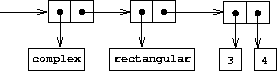In the above packages, we used add-rat, add-complex, and the other arithmetic procedures exactly as originally written. Once these definitions are internal to different installation procedures, however, they no longer need names that are distinct from each other: we could simply name them add, sub, mul, and div in both packages.

Exercise 2.77.  Louis Reasoner tries to evaluate the expression (magnitude z) where z is the object shown in figure 2.24. To his surprise, instead of the answer 5 he gets an error message from apply-generic, saying there is no method for the operation magnitude on the types (complex). He shows this interaction to Alyssa P. Hacker, who says ``The problem is that the complex-number selectors were never defined for complex numbers, just for polar and rectangular numbers. All you have to do to make this work is add the following to the complex package:''

(put 'real-part '(complex) real-part)
(put 'imag-part '(complex) imag-part)
(put 'magnitude '(complex) magnitude)
(put 'angle '(complex) angle)

Describe in detail why this works. As an example, trace through all the procedures called in evaluating the expression (magnitude z) where z is the object shown in figure 2.24. In particular, how many times is apply-generic invoked? What procedure is dispatched to in each case?

Exercise 2.78.  The internal procedures in the scheme-number package are essentially nothing more than calls to the primitive procedures +, -, etc. It was not possible to use the primitives of the language directly because our type-tag system requires that each data object have a type attached to it. In fact, however, all Lisp implementations do have a type system, which they use internally. Primitive predicates such as symbol? and number? determine whether data objects have particular types. Modify the definitions of type-tag, contents, and attach-tag from section 2.4.2 so that our generic system takes advantage of Scheme's internal type system. That is to say, the system should work as before except that ordinary numbers should be represented simply as Scheme numbers rather than as pairs whose car is the symbol scheme-number.

Exercise 2.79.  Define a generic equality predicate equ? that tests the equality of two numbers, and install it in the generic arithmetic package. This operation should work for ordinary numbers, rational numbers, and complex numbers.

Exercise 2.80.  Define a generic predicate =zero? that tests if its argument is zero, and install it in the generic arithmetic package. This operation should work for ordinary numbers, rational numbers, and complex numbers.

### 2.5.2  Combining Data of Different Types

We have seen how to define a unified arithmetic system that encompasses ordinary numbers, complex numbers, rational numbers, and any other type of number we might decide to invent, but we have ignored an important issue. The operations we have defined so far treat the different data types as being completely independent. Thus, there are separate packages for adding, say, two ordinary numbers, or two complex numbers. What we have not yet considered is the fact that it is meaningful to define operations that cross the type boundaries, such as the addition of a complex number to an ordinary number. We have gone to great pains to introduce barriers between parts of our programs so that they can be developed and understood separately. We would like to introduce the cross-type operations in some carefully controlled way, so that we can support them without seriously violating our module boundaries.

One way to handle cross-type operations is to design a different procedure for each possible combination of types for which the operation is valid. For example, we could extend the complex-number package so that it provides a procedure for adding complex numbers to ordinary numbers and installs this in the table using the tag (complex scheme-number):49

;; to be included in the complex package
(make-from-real-imag (+ (real-part z) x)
(imag-part z)))

This technique works, but it is cumbersome. With such a system, the cost of introducing a new type is not just the construction of the package of procedures for that type but also the construction and installation of the procedures that implement the cross-type operations. This can easily be much more code than is needed to define the operations on the type itself. The method also undermines our ability to combine separate packages additively, or least to limit the extent to which the implementors of the individual packages need to take account of other packages. For instance, in the example above, it seems reasonable that handling mixed operations on complex numbers and ordinary numbers should be the responsibility of the complex-number package. Combining rational numbers and complex numbers, however, might be done by the complex package, by the rational package, or by some third package that uses operations extracted from these two packages. Formulating coherent policies on the division of responsibility among packages can be an overwhelming task in designing systems with many packages and many cross-type operations.

#### Coercion

In the general situation of completely unrelated operations acting on completely unrelated types, implementing explicit cross-type operations, cumbersome though it may be, is the best that one can hope for. Fortunately, we can usually do better by taking advantage of additional structure that may be latent in our type system. Often the different data types are not completely independent, and there may be ways by which objects of one type may be viewed as being of another type. This process is called coercion. For example, if we are asked to arithmetically combine an ordinary number with a complex number, we can view the ordinary number as a complex number whose imaginary part is zero. This transforms the problem to that of combining two complex numbers, which can be handled in the ordinary way by the complex-arithmetic package.

In general, we can implement this idea by designing coercion procedures that transform an object of one type into an equivalent object of another type. Here is a typical coercion procedure, which transforms a given ordinary number to a complex number with that real part and zero imaginary part:

(define (scheme-number->complex n)
(make-complex-from-real-imag (contents n) 0))

We install these coercion procedures in a special coercion table, indexed under the names of the two types:

(put-coercion 'scheme-number 'complex scheme-number->complex)

(We assume that there are put-coercion and get-coercion procedures available for manipulating this table.) Generally some of the slots in the table will be empty, because it is not generally possible to coerce an arbitrary data object of each type into all other types. For example, there is no way to coerce an arbitrary complex number to an ordinary number, so there will be no general complex->scheme-number procedure included in the table.

Once the coercion table has been set up, we can handle coercion in a uniform manner by modifying the apply-generic procedure of section 2.4.3. When asked to apply an operation, we first check whether the operation is defined for the arguments' types, just as before. If so, we dispatch to the procedure found in the operation-and-type table. Otherwise, we try coercion. For simplicity, we consider only the case where there are two arguments.50 We check the coercion table to see if objects of the first type can be coerced to the second type. If so, we coerce the first argument and try the operation again. If objects of the first type cannot in general be coerced to the second type, we try the coercion the other way around to see if there is a way to coerce the second argument to the type of the first argument. Finally, if there is no known way to coerce either type to the other type, we give up. Here is the procedure:

(define (apply-generic op . args)
(let ((type-tags (map type-tag args)))
(let ((proc (get op type-tags)))
(if proc
(apply proc (map contents args))
(if (= (length args) 2)
(let ((type1 (car type-tags))
(a1 (car args))
(let ((t1->t2 (get-coercion type1 type2))
(t2->t1 (get-coercion type2 type1)))
(cond (t1->t2
(apply-generic op (t1->t2 a1) a2))
(t2->t1
(apply-generic op a1 (t2->t1 a2)))
(else
(error "No method for these types"
(list op type-tags))))))
(error "No method for these types"
(list op type-tags)))))))

This coercion scheme has many advantages over the method of defining explicit cross-type operations, as outlined above. Although we still need to write coercion procedures to relate the types (possibly n2 procedures for a system with n types), we need to write only one procedure for each pair of types rather than a different procedure for each collection of types and each generic operation.51 What we are counting on here is the fact that the appropriate transformation between types depends only on the types themselves, not on the operation to be applied.

On the other hand, there may be applications for which our coercion scheme is not general enough. Even when neither of the objects to be combined can be converted to the type of the other it may still be possible to perform the operation by converting both objects to a third type. In order to deal with such complexity and still preserve modularity in our programs, it is usually necessary to build systems that take advantage of still further structure in the relations among types, as we discuss next.

#### Hierarchies of types

The coercion scheme presented above relied on the existence of natural relations between pairs of types. Often there is more ``global'' structure in how the different types relate to each other. For instance, suppose we are building a generic arithmetic system to handle integers, rational numbers, real numbers, and complex numbers. In such a system, it is quite natural to regard an integer as a special kind of rational number, which is in turn a special kind of real number, which is in turn a special kind of complex number. What we actually have is a so-called hierarchy of types, in which, for example, integers are a subtype of rational numbers (i.e., any operation that can be applied to a rational number can automatically be applied to an integer). Conversely, we say that rational numbers form a supertype of integers. The particular hierarchy we have here is of a very simple kind, in which each type has at most one supertype and at most one subtype. Such a structure, called a tower, is illustrated in figure 2.25.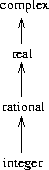If we have a tower structure, then we can greatly simplify the problem of adding a new type to the hierarchy, for we need only specify how the new type is embedded in the next supertype above it and how it is the supertype of the type below it. For example, if we want to add an integer to a complex number, we need not explicitly define a special coercion procedure integer->complex. Instead, we define how an integer can be transformed into a rational number, how a rational number is transformed into a real number, and how a real number is transformed into a complex number. We then allow the system to transform the integer into a complex number through these steps and then add the two complex numbers.

We can redesign our apply-generic procedure in the following way: For each type, we need to supply a raise procedure, which ``raises'' objects of that type one level in the tower. Then when the system is required to operate on objects of different types it can successively raise the lower types until all the objects are at the same level in the tower. (Exercises 2.83 and  2.84 concern the details of implementing such a strategy.)

Another advantage of a tower is that we can easily implement the notion that every type ``inherits'' all operations defined on a supertype. For instance, if we do not supply a special procedure for finding the real part of an integer, we should nevertheless expect that real-part will be defined for integers by virtue of the fact that integers are a subtype of complex numbers. In a tower, we can arrange for this to happen in a uniform way by modifying apply-generic. If the required operation is not directly defined for the type of the object given, we raise the object to its supertype and try again. We thus crawl up the tower, transforming our argument as we go, until we either find a level at which the desired operation can be performed or hit the top (in which case we give up).

Yet another advantage of a tower over a more general hierarchy is that it gives us a simple way to ``lower'' a data object to the simplest representation. For example, if we add 2 + 3i to 4 - 3i, it would be nice to obtain the answer as the integer 6 rather than as the complex number 6 + 0i. Exercise 2.85 discusses a way to implement such a lowering operation. (The trick is that we need a general way to distinguish those objects that can be lowered, such as 6 + 0i, from those that cannot, such as 6 + 2i.)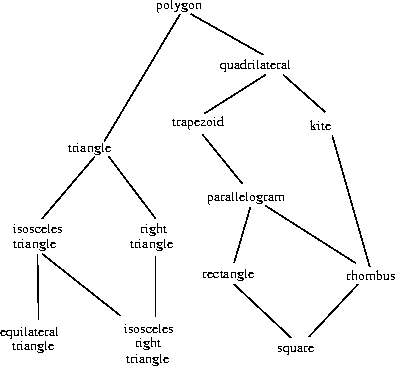If the data types in our system can be naturally arranged in a tower, this greatly simplifies the problems of dealing with generic operations on different types, as we have seen. Unfortunately, this is usually not the case. Figure 2.26 illustrates a more complex arrangement of mixed types, this one showing relations among different types of geometric figures. We see that, in general, a type may have more than one subtype. Triangles and quadrilaterals, for instance, are both subtypes of polygons. In addition, a type may have more than one supertype. For example, an isosceles right triangle may be regarded either as an isosceles triangle or as a right triangle. This multiple-supertypes issue is particularly thorny, since it means that there is no unique way to ``raise'' a type in the hierarchy. Finding the ``correct'' supertype in which to apply an operation to an object may involve considerable searching through the entire type network on the part of a procedure such as apply-generic. Since there generally are multiple subtypes for a type, there is a similar problem in coercing a value ``down'' the type hierarchy. Dealing with large numbers of interrelated types while still preserving modularity in the design of large systems is very difficult, and is an area of much current research.52

Exercise 2.81.  Louis Reasoner has noticed that apply-generic may try to coerce the arguments to each other's type even if they already have the same type. Therefore, he reasons, we need to put procedures in the coercion table to "coerce" arguments of each type to their own type. For example, in addition to the scheme-number->complex coercion shown above, he would do:

(define (scheme-number->scheme-number n) n)
(define (complex->complex z) z)
(put-coercion 'scheme-number 'scheme-number
scheme-number->scheme-number)
(put-coercion 'complex 'complex complex->complex)

a. With Louis's coercion procedures installed, what happens if apply-generic is called with two arguments of type scheme-number or two arguments of type complex for an operation that is not found in the table for those types? For example, assume that we've defined a generic exponentiation operation:

(define (exp x y) (apply-generic 'exp x y))

and have put a procedure for exponentiation in the Scheme-number package but not in any other package:

;; following added to Scheme-number package
(put 'exp '(scheme-number scheme-number)
(lambda (x y) (tag (expt x y)))) ; using primitive expt

What happens if we call exp with two complex numbers as arguments?

b. Is Louis correct that something had to be done about coercion with arguments of the same type, or does apply-generic work correctly as is?

c. Modify apply-generic so that it doesn't try coercion if the two arguments have the same type.

Exercise 2.82.  Show how to generalize apply-generic to handle coercion in the general case of multiple arguments. One strategy is to attempt to coerce all the arguments to the type of the first argument, then to the type of the second argument, and so on. Give an example of a situation where this strategy (and likewise the two-argument version given above) is not sufficiently general. (Hint: Consider the case where there are some suitable mixed-type operations present in the table that will not be tried.)

Exercise 2.83.  Suppose you are designing a generic arithmetic system for dealing with the tower of types shown in figure 2.25: integer, rational, real, complex. For each type (except complex), design a procedure that raises objects of that type one level in the tower. Show how to install a generic raise operation that will work for each type (except complex).

Exercise 2.84.  Using the raise operation of exercise 2.83, modify the apply-generic procedure so that it coerces its arguments to have the same type by the method of successive raising, as discussed in this section. You will need to devise a way to test which of two types is higher in the tower. Do this in a manner that is ``compatible'' with the rest of the system and will not lead to problems in adding new levels to the tower.

Exercise 2.85.  This section mentioned a method for ``simplifying'' a data object by lowering it in the tower of types as far as possible. Design a procedure drop that accomplishes this for the tower described in exercise 2.83. The key is to decide, in some general way, whether an object can be lowered. For example, the complex number 1.5 + 0i can be lowered as far as real, the complex number 1 + 0i can be lowered as far as integer, and the complex number 2 + 3i cannot be lowered at all. Here is a plan for determining whether an object can be lowered: Begin by defining a generic operation project that ``pushes'' an object down in the tower. For example, projecting a complex number would involve throwing away the imaginary part. Then a number can be dropped if, when we project it and raise the result back to the type we started with, we end up with something equal to what we started with. Show how to implement this idea in detail, by writing a drop procedure that drops an object as far as possible. You will need to design the various projection operations53 and install project as a generic operation in the system. You will also need to make use of a generic equality predicate, such as described in exercise 2.79. Finally, use drop to rewrite apply-generic from exercise 2.84 so that it ``simplifies'' its answers.

Exercise 2.86.  Suppose we want to handle complex numbers whose real parts, imaginary parts, magnitudes, and angles can be either ordinary numbers, rational numbers, or other numbers we might wish to add to the system. Describe and implement the changes to the system needed to accommodate this. You will have to define operations such as sine and cosine that are generic over ordinary numbers and rational numbers.

### 2.5.3  Example: Symbolic Algebra

The manipulation of symbolic algebraic expressions is a complex process that illustrates many of the hardest problems that occur in the design of large-scale systems. An algebraic expression, in general, can be viewed as a hierarchical structure, a tree of operators applied to operands. We can construct algebraic expressions by starting with a set of primitive objects, such as constants and variables, and combining these by means of algebraic operators, such as addition and multiplication. As in other languages, we form abstractions that enable us to refer to compound objects in simple terms. Typical abstractions in symbolic algebra are ideas such as linear combination, polynomial, rational function, or trigonometric function. We can regard these as compound ``types,'' which are often useful for directing the processing of expressions. For example, we could describe the expression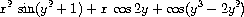as a polynomial in x with coefficients that are trigonometric functions of polynomials in y whose coefficients are integers.

We will not attempt to develop a complete algebraic-manipulation system here. Such systems are exceedingly complex programs, embodying deep algebraic knowledge and elegant algorithms. What we will do is look at a simple but important part of algebraic manipulation: the arithmetic of polynomials. We will illustrate the kinds of decisions the designer of such a system faces, and how to apply the ideas of abstract data and generic operations to help organize this effort.

#### Arithmetic on polynomials

Our first task in designing a system for performing arithmetic on polynomials is to decide just what a polynomial is. Polynomials are normally defined relative to certain variables (the indeterminates of the polynomial). For simplicity, we will restrict ourselves to polynomials having just one indeterminate (univariate polynomials).54 We will define a polynomial to be a sum of terms, each of which is either a coefficient, a power of the indeterminate, or a product of a coefficient and a power of the indeterminate. A coefficient is defined as an algebraic expression that is not dependent upon the indeterminate of the polynomial. For example,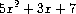is a simple polynomial in x, and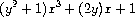is a polynomial in x whose coefficients are polynomials in y.

Already we are skirting some thorny issues. Is the first of these polynomials the same as the polynomial 5y2 + 3y + 7, or not? A reasonable answer might be ``yes, if we are considering a polynomial purely as a mathematical function, but no, if we are considering a polynomial to be a syntactic form.'' The second polynomial is algebraically equivalent to a polynomial in y whose coefficients are polynomials in x. Should our system recognize this, or not? Furthermore, there are other ways to represent a polynomial -- for example, as a product of factors, or (for a univariate polynomial) as the set of roots, or as a listing of the values of the polynomial at a specified set of points.55 We can finesse these questions by deciding that in our algebraic-manipulation system a ``polynomial'' will be a particular syntactic form, not its underlying mathematical meaning.

Now we must consider how to go about doing arithmetic on polynomials. In this simple system, we will consider only addition and multiplication. Moreover, we will insist that two polynomials to be combined must have the same indeterminate.

We will approach the design of our system by following the familiar discipline of data abstraction. We will represent polynomials using a data structure called a poly, which consists of a variable and a collection of terms. We assume that we have selectors variable and term-list that extract those parts from a poly and a constructor make-poly that assembles a poly from a given variable and a term list. A variable will be just a symbol, so we can use the same-variable? procedure of section 2.3.2 to compare variables. The following procedures define addition and multiplication of polys:

(if (same-variable? (variable p1) (variable p2))
(make-poly (variable p1)
(term-list p2)))
(error "Polys not in same var -- ADD-POLY"
(list p1 p2))))
(define (mul-poly p1 p2)
(if (same-variable? (variable p1) (variable p2))
(make-poly (variable p1)
(mul-terms (term-list p1)
(term-list p2)))
(error "Polys not in same var -- MUL-POLY"
(list p1 p2))))

To incorporate polynomials into our generic arithmetic system, we need to supply them with type tags. We'll use the tag polynomial, and install appropriate operations on tagged polynomials in the operation table. We'll embed all our code in an installation procedure for the polynomial package, similar to the ones in section 2.5.1:

(define (install-polynomial-package)
;; internal procedures
;; representation of poly
(define (make-poly variable term-list)
(cons variable term-list))
(define (variable p) (car p))
(define (term-list p) (cdr p))
<procedures same-variable? and variable? from section 2.3.2>
;; representation of terms and term lists
<procedures adjoin-term ...coeff from text below>

;; continued on next page

(define (mul-poly p1 p2) ...)
<procedures used by mul-poly>
;; interface to rest of the system
(define (tag p) (attach-tag 'polynomial p))
(lambda (p1 p2) (tag (add-poly p1 p2))))
(put 'mul '(polynomial polynomial)
(lambda (p1 p2) (tag (mul-poly p1 p2))))
(put 'make 'polynomial
(lambda (var terms) (tag (make-poly var terms))))
'done)

Polynomial addition is performed termwise. Terms of the same order (i.e., with the same power of the indeterminate) must be combined. This is done by forming a new term of the same order whose coefficient is the sum of the coefficients of the addends. Terms in one addend for which there are no terms of the same order in the other addend are simply accumulated into the sum polynomial being constructed.

In order to manipulate term lists, we will assume that we have a constructor the-empty-termlist that returns an empty term list and a constructor adjoin-term that adjoins a new term to a term list. We will also assume that we have a predicate empty-termlist? that tells if a given term list is empty, a selector first-term that extracts the highest-order term from a term list, and a selector rest-terms that returns all but the highest-order term. To manipulate terms, we will suppose that we have a constructor make-term that constructs a term with given order and coefficient, and selectors order and coeff that return, respectively, the order and the coefficient of the term. These operations allow us to consider both terms and term lists as data abstractions, whose concrete representations we can worry about separately.

Here is the procedure that constructs the term list for the sum of two polynomials:56

(cond ((empty-termlist? L1) L2)
((empty-termlist? L2) L1)
(else
(let ((t1 (first-term L1)) (t2 (first-term L2)))
(cond ((> (order t1) (order t2))
((< (order t1) (order t2))
(else
(make-term (order t1)
(rest-terms L2)))))))))

The most important point to note here is that we used the generic addition procedure add to add together the coefficients of the terms being combined. This has powerful consequences, as we will see below.

In order to multiply two term lists, we multiply each term of the first list by all the terms of the other list, repeatedly using mul-term-by-all-terms, which multiplies a given term by all terms in a given term list. The resulting term lists (one for each term of the first list) are accumulated into a sum. Multiplying two terms forms a term whose order is the sum of the orders of the factors and whose coefficient is the product of the coefficients of the factors:

(define (mul-terms L1 L2)
(if (empty-termlist? L1)
(the-empty-termlist)
(mul-terms (rest-terms L1) L2))))
(define (mul-term-by-all-terms t1 L)
(if (empty-termlist? L)
(the-empty-termlist)
(let ((t2 (first-term L)))
(make-term (+ (order t1) (order t2))
(mul (coeff t1) (coeff t2)))
(mul-term-by-all-terms t1 (rest-terms L))))))

This is really all there is to polynomial addition and multiplication. Notice that, since we operate on terms using the generic procedures add and mul, our polynomial package is automatically able to handle any type of coefficient that is known about by the generic arithmetic package. If we include a coercion mechanism such as one of those discussed in section 2.5.2, then we also are automatically able to handle operations on polynomials of different coefficient types, such as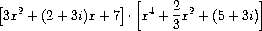Because we installed the polynomial addition and multiplication procedures add-poly and mul-poly in the generic arithmetic system as the add and mul operations for type polynomial, our system is also automatically able to handle polynomial operations such as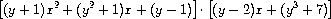The reason is that when the system tries to combine coefficients, it will dispatch through add and mul. Since the coefficients are themselves polynomials (in y), these will be combined using add-poly and mul-poly. The result is a kind of ``data-directed recursion'' in which, for example, a call to mul-poly will result in recursive calls to mul-poly in order to multiply the coefficients. If the coefficients of the coefficients were themselves polynomials (as might be used to represent polynomials in three variables), the data direction would ensure that the system would follow through another level of recursive calls, and so on through as many levels as the structure of the data dictates.57

#### Representing term lists

Finally, we must confront the job of implementing a good representation for term lists. A term list is, in effect, a set of coefficients keyed by the order of the term. Hence, any of the methods for representing sets, as discussed in section 2.3.3, can be applied to this task. On the other hand, our procedures add-terms and mul-terms always access term lists sequentially from highest to lowest order. Thus, we will use some kind of ordered list representation.

How should we structure the list that represents a term list? One consideration is the ``density'' of the polynomials we intend to manipulate. A polynomial is said to be dense if it has nonzero coefficients in terms of most orders. If it has many zero terms it is said to be sparse. For example,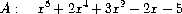is a dense polynomial, whereas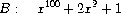is sparse.

The term lists of dense polynomials are most efficiently represented as lists of the coefficients. For example, A above would be nicely represented as (1 2 0 3 -2 -5). The order of a term in this representation is the length of the sublist beginning with that term's coefficient, decremented by 1.58 This would be a terrible representation for a sparse polynomial such as B: There would be a giant list of zeros punctuated by a few lonely nonzero terms. A more reasonable representation of the term list of a sparse polynomial is as a list of the nonzero terms, where each term is a list containing the order of the term and the coefficient for that order. In such a scheme, polynomial B is efficiently represented as ((100 1) (2 2) (0 1)). As most polynomial manipulations are performed on sparse polynomials, we will use this method. We will assume that term lists are represented as lists of terms, arranged from highest-order to lowest-order term. Once we have made this decision, implementing the selectors and constructors for terms and term lists is straightforward:59

(if (=zero? (coeff term))
term-list
(cons term term-list)))
(define (the-empty-termlist) '())
(define (first-term term-list) (car term-list))
(define (rest-terms term-list) (cdr term-list))
(define (empty-termlist? term-list) (null? term-list))
(define (make-term order coeff) (list order coeff))
(define (order term) (car term))

where =zero? is as defined in exercise 2.80. (See also exercise 2.87 below.)

Users of the polynomial package will create (tagged) polynomials by means of the procedure:

(define (make-polynomial var terms)
((get 'make 'polynomial) var terms))

Exercise 2.87.  Install =zero? for polynomials in the generic arithmetic package. This will allow adjoin-term to work for polynomials with coefficients that are themselves polynomials.

Exercise 2.88.  Extend the polynomial system to include subtraction of polynomials. (Hint: You may find it helpful to define a generic negation operation.)

Exercise 2.89.  Define procedures that implement the term-list representation described above as appropriate for dense polynomials.

Exercise 2.90.  Suppose we want to have a polynomial system that is efficient for both sparse and dense polynomials. One way to do this is to allow both kinds of term-list representations in our system. The situation is analogous to the complex-number example of section 2.4, where we allowed both rectangular and polar representations. To do this we must distinguish different types of term lists and make the operations on term lists generic. Redesign the polynomial system to implement this generalization. This is a major effort, not a local change.

Exercise 2.91.  A univariate polynomial can be divided by another one to produce a polynomial quotient and a polynomial remainder. For example,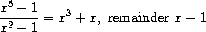Division can be performed via long division. That is, divide the highest-order term of the dividend by the highest-order term of the divisor. The result is the first term of the quotient. Next, multiply the result by the divisor, subtract that from the dividend, and produce the rest of the answer by recursively dividing the difference by the divisor. Stop when the order of the divisor exceeds the order of the dividend and declare the dividend to be the remainder. Also, if the dividend ever becomes zero, return zero as both quotient and remainder.

We can design a div-poly procedure on the model of add-poly and mul-poly. The procedure checks to see if the two polys have the same variable. If so, div-poly strips off the variable and passes the problem to div-terms, which performs the division operation on term lists. Div-poly finally reattaches the variable to the result supplied by div-terms. It is convenient to design div-terms to compute both the quotient and the remainder of a division. Div-terms can take two term lists as arguments and return a list of the quotient term list and the remainder term list.

Complete the following definition of div-terms by filling in the missing expressions. Use this to implement div-poly, which takes two polys as arguments and returns a list of the quotient and remainder polys.

(define (div-terms L1 L2)
(if (empty-termlist? L1)
(list (the-empty-termlist) (the-empty-termlist))
(let ((t1 (first-term L1))
(t2 (first-term L2)))
(if (> (order t2) (order t1))
(list (the-empty-termlist) L1)
(let ((new-c (div (coeff t1) (coeff t2)))
(new-o (- (order t1) (order t2))))
(let ((rest-of-result
<compute rest of result recursively>
))
<form complete result>
))))))

#### Hierarchies of types in symbolic algebra

Our polynomial system illustrates how objects of one type (polynomials) may in fact be complex objects that have objects of many different types as parts. This poses no real difficulty in defining generic operations. We need only install appropriate generic operations for performing the necessary manipulations of the parts of the compound types. In fact, we saw that polynomials form a kind of ``recursive data abstraction,'' in that parts of a polynomial may themselves be polynomials. Our generic operations and our data-directed programming style can handle this complication without much trouble.

On the other hand, polynomial algebra is a system for which the data types cannot be naturally arranged in a tower. For instance, it is possible to have polynomials in x whose coefficients are polynomials in y. It is also possible to have polynomials in y whose coefficients are polynomials in x. Neither of these types is ``above'' the other in any natural way, yet it is often necessary to add together elements from each set. There are several ways to do this. One possibility is to convert one polynomial to the type of the other by expanding and rearranging terms so that both polynomials have the same principal variable. One can impose a towerlike structure on this by ordering the variables and thus always converting any polynomial to a ``canonical form'' with the highest-priority variable dominant and the lower-priority variables buried in the coefficients. This strategy works fairly well, except that the conversion may expand a polynomial unnecessarily, making it hard to read and perhaps less efficient to work with. The tower strategy is certainly not natural for this domain or for any domain where the user can invent new types dynamically using old types in various combining forms, such as trigonometric functions, power series, and integrals.

It should not be surprising that controlling coercion is a serious problem in the design of large-scale algebraic-manipulation systems. Much of the complexity of such systems is concerned with relationships among diverse types. Indeed, it is fair to say that we do not yet completely understand coercion. In fact, we do not yet completely understand the concept of a data type. Nevertheless, what we know provides us with powerful structuring and modularity principles to support the design of large systems.

Exercise 2.92.  By imposing an ordering on variables, extend the polynomial package so that addition and multiplication of polynomials works for polynomials in different variables. (This is not easy!)

#### Extended exercise: Rational functions

We can extend our generic arithmetic system to include rational functions. These are ``fractions'' whose numerator and denominator are polynomials, such as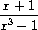The system should be able to add, subtract, multiply, and divide rational functions, and to perform such computations as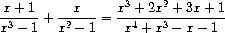(Here the sum has been simplified by removing common factors. Ordinary ``cross multiplication'' would have produced a fourth-degree polynomial over a fifth-degree polynomial.)

If we modify our rational-arithmetic package so that it uses generic operations, then it will do what we want, except for the problem of reducing fractions to lowest terms.

Exercise 2.93.  Modify the rational-arithmetic package to use generic operations, but change make-rat so that it does not attempt to reduce fractions to lowest terms. Test your system by calling make-rational on two polynomials to produce a rational function

(define p1 (make-polynomial 'x '((2 1)(0 1))))
(define p2 (make-polynomial 'x '((3 1)(0 1))))
(define rf (make-rational p2 p1))

Now add rf to itself, using add. You will observe that this addition procedure does not reduce fractions to lowest terms.

We can reduce polynomial fractions to lowest terms using the same idea we used with integers: modifying make-rat to divide both the numerator and the denominator by their greatest common divisor. The notion of ``greatest common divisor'' makes sense for polynomials. In fact, we can compute the GCD of two polynomials using essentially the same Euclid's Algorithm that works for integers.60 The integer version is

(define (gcd a b)
(if (= b 0)
a
(gcd b (remainder a b))))

Using this, we could make the obvious modification to define a GCD operation that works on term lists:

(define (gcd-terms a b)
(if (empty-termlist? b)
a
(gcd-terms b (remainder-terms a b))))

where remainder-terms picks out the remainder component of the list returned by the term-list division operation div-terms that was implemented in exercise 2.91.

Exercise 2.94.  Using div-terms, implement the procedure remainder-terms and use this to define gcd-terms as above. Now write a procedure gcd-poly that computes the polynomial GCD of two polys. (The procedure should signal an error if the two polys are not in the same variable.) Install in the system a generic operation greatest-common-divisor that reduces to gcd-poly for polynomials and to ordinary gcd for ordinary numbers. As a test, try

(define p1 (make-polynomial 'x '((4 1) (3 -1) (2 -2) (1 2))))
(define p2 (make-polynomial 'x '((3 1) (1 -1))))
(greatest-common-divisor p1 p2)

and check your result by hand.

Exercise 2.95.  Define P1, P2, and P3 to be the polynomials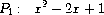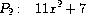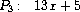Now define Q1 to be the product of P1 and P2 and Q2 to be the product of P1 and P3, and use greatest-common-divisor (exercise 2.94) to compute the GCD of Q1 and Q2. Note that the answer is not the same as P1. This example introduces noninteger operations into the computation, causing difficulties with the GCD algorithm.61 To understand what is happening, try tracing gcd-terms while computing the GCD or try performing the division by hand.

We can solve the problem exhibited in exercise 2.95 if we use the following modification of the GCD algorithm (which really works only in the case of polynomials with integer coefficients). Before performing any polynomial division in the GCD computation, we multiply the dividend by an integer constant factor, chosen to guarantee that no fractions will arise during the division process. Our answer will thus differ from the actual GCD by an integer constant factor, but this does not matter in the case of reducing rational functions to lowest terms; the GCD will be used to divide both the numerator and denominator, so the integer constant factor will cancel out.

More precisely, if P and Q are polynomials, let O1 be the order of P (i.e., the order of the largest term of P) and let O2 be the order of Q. Let c be the leading coefficient of Q. Then it can be shown that, if we multiply P by the integerizing factor c1+O1 -O2, the resulting polynomial can be divided by Q by using the div-terms algorithm without introducing any fractions. The operation of multiplying the dividend by this constant and then dividing is sometimes called the pseudodivision of P by Q. The remainder of the division is called the pseudoremainder.

Exercise 2.96.  a.    Implement the procedure pseudoremainder-terms, which is just like remainder-terms except that it multiplies the dividend by the integerizing factor described above before calling div-terms. Modify gcd-terms to use pseudoremainder-terms, and verify that greatest-common-divisor now produces an answer with integer coefficients on the example in exercise 2.95.

b.    The GCD now has integer coefficients, but they are larger than those of P1. Modify gcd-terms so that it removes common factors from the coefficients of the answer by dividing all the coefficients by their (integer) greatest common divisor.

Thus, here is how to reduce a rational function to lowest terms:

• Compute the GCD of the numerator and denominator, using the version of gcd-terms from exercise 2.96.

• When you obtain the GCD, multiply both numerator and denominator by the same integerizing factor before dividing through by the GCD, so that division by the GCD will not introduce any noninteger coefficients. As the factor you can use the leading coefficient of the GCD raised to the power 1 + O1 - O2, where O2 is the order of the GCD and O1 is the maximum of the orders of the numerator and denominator. This will ensure that dividing the numerator and denominator by the GCD will not introduce any fractions.

• The result of this operation will be a numerator and denominator with integer coefficients. The coefficients will normally be very large because of all of the integerizing factors, so the last step is to remove the redundant factors by computing the (integer) greatest common divisor of all the coefficients of the numerator and the denominator and dividing through by this factor.

Exercise 2.97.  a. Implement this algorithm as a procedure reduce-terms that takes two term lists n and d as arguments and returns a list nn, dd, which are n and d reduced to lowest terms via the algorithm given above. Also write a procedure reduce-poly, analogous to add-poly, that checks to see if the two polys have the same variable. If so, reduce-poly strips off the variable and passes the problem to reduce-terms, then reattaches the variable to the two term lists supplied by reduce-terms.

b. Define a procedure analogous to reduce-terms that does what the original make-rat did for integers:

(define (reduce-integers n d)
(let ((g (gcd n d)))
(list (/ n g) (/ d g))))

and define reduce as a generic operation that calls apply-generic to dispatch to either reduce-poly (for polynomial arguments) or reduce-integers (for scheme-number arguments). You can now easily make the rational-arithmetic package reduce fractions to lowest terms by having make-rat call reduce before combining the given numerator and denominator to form a rational number. The system now handles rational expressions in either integers or polynomials. To test your program, try the example at the beginning of this extended exercise:

(define p1 (make-polynomial 'x '((1 1)(0 1))))
(define p2 (make-polynomial 'x '((3 1)(0 -1))))
(define p3 (make-polynomial 'x '((1 1))))
(define p4 (make-polynomial 'x '((2 1)(0 -1))))

(define rf1 (make-rational p1 p2))
(define rf2 (make-rational p3 p4))

See if you get the correct answer, correctly reduced to lowest terms.

The GCD computation is at the heart of any system that does operations on rational functions. The algorithm used above, although mathematically straightforward, is extremely slow. The slowness is due partly to the large number of division operations and partly to the enormous size of the intermediate coefficients generated by the pseudodivisions. One of the active areas in the development of algebraic-manipulation systems is the design of better algorithms for computing polynomial GCDs.62

49 We also have to supply an almost identical procedure to handle the types (scheme-number complex).

50 See exercise 2.82 for generalizations.

51 If we are clever, we can usually get by with fewer than n2 coercion procedures. For instance, if we know how to convert from type 1 to type 2 and from type 2 to type 3, then we can use this knowledge to convert from type 1 to type 3. This can greatly decrease the number of coercion procedures we need to supply explicitly when we add a new type to the system. If we are willing to build the required amount of sophistication into our system, we can have it search the ``graph'' of relations among types and automatically generate those coercion procedures that can be inferred from the ones that are supplied explicitly.

52 This statement, which also appears in the first edition of this book, is just as true now as it was when we wrote it twelve years ago. Developing a useful, general framework for expressing the relations among different types of entities (what philosophers call ``ontology'') seems intractably difficult. The main difference between the confusion that existed ten years ago and the confusion that exists now is that now a variety of inadequate ontological theories have been embodied in a plethora of correspondingly inadequate programming languages. For example, much of the complexity of object-oriented programming languages -- and the subtle and confusing differences among contemporary object-oriented languages -- centers on the treatment of generic operations on interrelated types. Our own discussion of computational objects in chapter 3 avoids these issues entirely. Readers familiar with object-oriented programming will notice that we have much to say in chapter 3 about local state, but we do not even mention ``classes'' or ``inheritance.'' In fact, we suspect that these problems cannot be adequately addressed in terms of computer-language design alone, without also drawing on work in knowledge representation and automated reasoning.

53 A real number can be projected to an integer using the round primitive, which returns the closest integer to its argument.

54 On the other hand, we will allow polynomials whose coefficients are themselves polynomials in other variables. This will give us essentially the same representational power as a full multivariate system, although it does lead to coercion problems, as discussed below.

55 For univariate polynomials, giving the value of a polynomial at a given set of points can be a particularly good representation. This makes polynomial arithmetic extremely simple. To obtain, for example, the sum of two polynomials represented in this way, we need only add the values of the polynomials at corresponding points. To transform back to a more familiar representation, we can use the Lagrange interpolation formula, which shows how to recover the coefficients of a polynomial of degree n given the values of the polynomial at n + 1 points.

56 This operation is very much like the ordered union-set operation we developed in exercise  2.62. In fact, if we think of the terms of the polynomial as a set ordered according to the power of the indeterminate, then the program that produces the term list for a sum is almost identical to union-set.

57 To make this work completely smoothly, we should also add to our generic arithmetic system the ability to coerce a ``number'' to a polynomial by regarding it as a polynomial of degree zero whose coefficient is the number. This is necessary if we are going to perform operations such as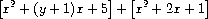which requires adding the coefficient y + 1 to the coefficient 2.

58 In these polynomial examples, we assume that we have implemented the generic arithmetic system using the type mechanism suggested in exercise 2.78. Thus, coefficients that are ordinary numbers will be represented as the numbers themselves rather than as pairs whose car is the symbol scheme-number.

59 Although we are assuming that term lists are ordered, we have implemented adjoin-term to simply cons the new term onto the existing term list. We can get away with this so long as we guarantee that the procedures (such as add-terms) that use adjoin-term always call it with a higher-order term than appears in the list. If we did not want to make such a guarantee, we could have implemented adjoin-term to be similar to the adjoin-set constructor for the ordered-list representation of sets (exercise 2.61).

60 The fact that Euclid's Algorithm works for polynomials is formalized in algebra by saying that polynomials form a kind of algebraic domain called a Euclidean ring. A Euclidean ring is a domain that admits addition, subtraction, and commutative multiplication, together with a way of assigning to each element x of the ring a positive integer ``measure'' m(x) with the properties that m(xy)> m(x) for any nonzero x and y and that, given any x and y, there exists a q such that y = qx + r and either r = 0 or m(r)< m(x). From an abstract point of view, this is what is needed to prove that Euclid's Algorithm works. For the domain of integers, the measure m of an integer is the absolute value of the integer itself. For the domain of polynomials, the measure of a polynomial is its degree.

61 In an implementation like MIT Scheme, this produces a polynomial that is indeed a divisor of Q1 and Q2, but with rational coefficients. In many other Scheme systems, in which division of integers can produce limited-precision decimal numbers, we may fail to get a valid divisor.

62 One extremely efficient and elegant method for computing polynomial GCDs was discovered by Richard Zippel (1979). The method is a probabilistic algorithm, as is the fast test for primality that we discussed in chapter 1. Zippel's book (1993) describes this method, together with other ways to compute polynomial GCDs.